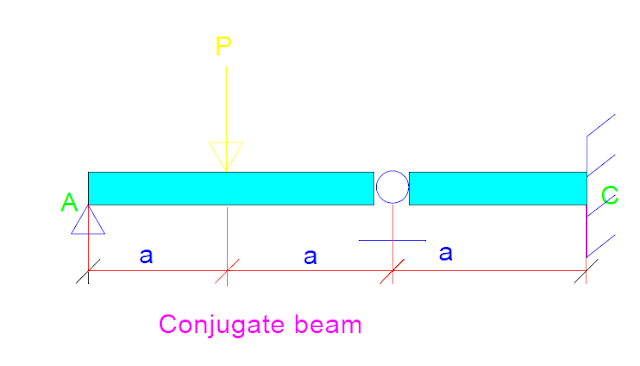### Conjugate beam Problem no:1 (beam with pin and internal roller)

Problem no:1, Determine the slope and displacement for the following beam at point C?

Figure 1

In this example, the pin support will stay the same in the conjugate beam. While the roller will change to hinge and the free end to a fixed support. So the conjugate beam will be the same as shown in figure no:2.Figure 2

Now we need to determine the moment diagram for the real beam.

First step is to determine the force at support A and B by the use of equilibrium equations:

Figure 3

Now we can draw the moment diagram for the real beam. To draw the moment diagram, we can draw the shear first, and the moment diagram will be the area of shear. The moment diagram for the real beam will be, as shown in figure no:4.

Figure 4

After drawing the shear and moment diagram, our conjugate beam will be loaded with the M/EI diagram of the real beam, as shown in figure no:5.

now to determine slope and displacement at c we need to calculate the shear moment at c by the use of equilibrium equations:
To simplify the solution, we will divide the beam into two sections, and we will start with section A, section A showed in figure no:6. as we know, the internal hinge carries only force and no moment

Section A

Figure 6 (section A)

Now, after determining Fb, we go to Section B to determine FC and MC.